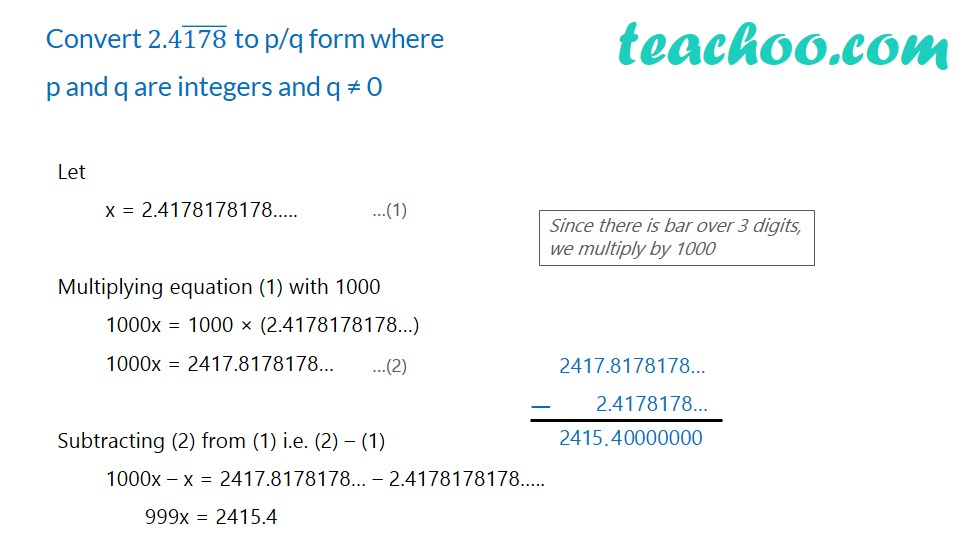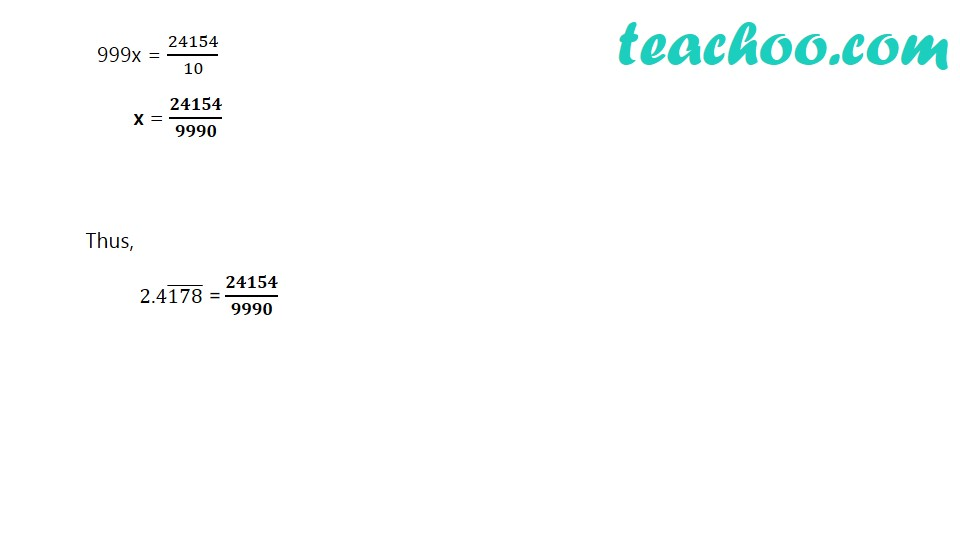Expressing decimal in p/q

Chapter 1 Class 9 Number Systems
Concept wiseLearn in your speed, with individual attention - Teachoo Maths 1-on-1 Class

### Transcript

Convert 2.4(178) ̅ to p/q form where p and q are integers and q ≠ 0 Let x = 2.4178178178….. Multiplying equation (1) with 1000 1000x = 1000 × (2.4178178178…) 1000x = 2417.8178178… Subtracting (2) from (1) i.e. (2) – (1) 1000x – x = 2417.8178178… – 2.4178178178….. 999x = 2415.4 Since there is bar over 3 digits, we multiply by 1000 2417.8178178… 2.4178178… 999x = 24154/10 x = 𝟐𝟒𝟏𝟓𝟒/𝟗𝟗𝟗𝟎 Thus, 2.4(178) ̅ = 𝟐𝟒𝟏𝟓𝟒/𝟗𝟗𝟗𝟎